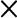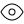Fixed Deposit up to 9.05% p.a.* Interest rates. Invest Now

01-01-2023 00:13:59

Recurring Deposit up to 8.50% p.a.* Interest rates. Invest Now

14-11-2022 14:09:00

Two Entities - One Shared Vision - STFC and SCUF are now merged as Shriram Finance

14-11-2022 14:08:45

# Premature Withdrawal of Fixed Deposit and its Effect on Interest Calculation

Posted: 26th September, 2022

Updated: 5th February, 2023

Written by -We may face sudden emergencies in our day-to-day lives that may affect our savings and lead us to either apply for a loan or break the investment funds. A Fixed Deposit (FD) is the most traditional savings option preferred by investors of all ages. However, premature withdrawal or breaking of an FD before its maturity incurs a penalty. The interest you earn on a fixed deposit investment is bound to decrease depending on the tenure you have selected for the deposit. Fixed depositminimal documentation if you already hold an account with banks/Non-Banking Financial Companies (NBFCs). An FD offers features like assured returns with high-interest rates at low market risk making it attractive to all investors.Investing in a Shriram Fixed Deposit gets you higher interest rates of up to 9.05%* p.a. per annum, including the additional interest of 0.50%* p.a. for senior citizens and 0.10%* p.a. for women depositors. You can use the Shriram FD calculator to determine the interest earned on the deposit upon maturity. Cumulative and non-cumulative FD plans are also available that allow you to customise the interest payout according to your requirement.Let's look at how a fixed deposit interest is calculated and understand what happens to the interest calculation if the deposit is prematurely withdrawn

## What is Premature Withdrawal of a Fixed Deposit?

When a depositor needs to close their fixed deposit before the maturity date, that is considered a premature withdrawal of an FD. A person may need to access the funds in their deposit during times of emergency. In such a case, the bank or NBFC will allow them to break the FD but will charge a penalty on both partial and whole withdrawal of the fixed deposit.The penalty charges depend on the deposit's tenure and the financial institution's guidelines.

## How is Interest Calculated on a Fixed Deposit?

Calculating the interest on a fixed deposit is easy and can be done manually or through an online FD calculator. There are two modes to calculate the interest on an FD: Simple interest and Compound interest.Simple interest is the interest earned on a fixed deposit with the principal amount alone. In contrast, compound interest is the interest earned on both the principal and interest amounts. Let us understand the calculations with respective formula and examples.

## Formula to Calculate Simple Interest:

Rate of interest per annum = R Simple interest can be calculated as principal x rate of interest x tenure divided by 100.If one invests Rs. 50,000 at 8% per annum for 5 years, the interest would be calculated like this-50,00 x 8 x 5 = 2,000,000.Thus, the simple interest you can get for a 5-year deposit is Rs. 20,000, and your maturity amount becomes Rs. 70,000.

## Formula to Calculate Compound Interest:

The compound interest is calculated with the principal amount and the interest earned in a year. The same formula is applicable, but the calculation will be done yearly.50,000 x 8 x 1 = 400,000.The interest earned for the first year would be Rs. 4000. This amount is added back to the principal, so the principal for the next year becomes Rs. 54,000 54,000 x 8 x 1 = 4,32,000.This way, the interest earned for the second year would be Rs. 4,320. This interest earned is then compounded yearly, making your maturity amount Rs. 73,457.The maturity amount will vary depending on the type of fixed deposit plan you choose. An easy way to check the interest rate and maturity amount is to choose Shriram FD calculator and start your investment journey.

## How does Premature FD Withdrawal Impact Interest Calculation?

When a person withdraws an FD before the maturity date, they will not receive the same rate of interest decided at the account's opening. The penalty charges range from 0.50%* p.a. to 1%, depending on the financial institution.There are two ways through which you can calculate the interest after the premature withdrawal of an FD. Let us understand better using cases.

• Case 1: If a person invests Rs. 1 lakh for 2 years at an interest rate of 7%. Let us suppose the interest rate for 1 year is 6.5%. After 1 year of the FD, the investor needs to close the deposit prematurely, and the bank or NBFC will re-calculate the interest at revised FD rates. The final FD rate would be 6.5% - 1% = 5.5%. The premature withdrawal amount will be calculated at the revised interest rate instead of the original 7%.
• Case 2: Let us assume that a person invests in an FD of Rs. 1 lakh for a tenure of 2 years at an interest rate of 6%. Assuming that the interest rate for 1 year is 7% at the time of booking, the penalty applied in the case of premature withdrawal is 1% of the effective interest rate. The interest rate earned for 1 Year is 6%. If you withdraw the FD after the completion of 1 year, the bank or NBFC will still calculate interest at the effective rate of 6% - 1% = 5% as the revised interest rate. Your premature withdrawal amount will be calculated at 5% instead of 6%.

Note: This example is only for indicative purposes. The actual calculation may differ at the withdrawal time and varies for every bank/NBFC.

## Continue your Financial Journey – Invest in Shriram Fixed Deposit

Although prematurely withdrawing a fixed deposit is easy, it is not advisable as you can be charged a penalty on your deposit. Furthermore, the interest is re-calculated and lowered according to the tenure you have completed with the deposit. You can always avoid breaking an FD by taking a loan A Shriram FD has a flexible tenure that you can use to plan your financial goals efficiently. In addition to higher interest rates, you also get an extra 0.25% interest on all matured Shriram Fixed Deposit renewals.

## FAQs

1. 1. What happens when you withdraw a fixed deposit early?

When a fixed deposit is withdrawn before the maturity date, you will be charged a penalty for breaking the deposit.

1. 2. Do we get the interest amount after the premature withdrawal of an FD?

You will get the accumulated interest upon prematurely withdrawing the fixed deposit. However, the interest is deducted as a penalty only if the FD has completed the tenure of a few months.

1. 3. How is the penalty on an FD calculated?

The penalty on an FD is typically charged at 0.50%* p.a. to 1% of the accumulated interest on your deposit.

1. 4. Can we withdraw a partial amount from FD?

Yes, a partial amount can be withdrawn from a fixed deposit if your bank or NBFC has a facility to allow it.

## Key Highlights:

• Premature withdrawal of a fixed deposit can be made easily, but it incurs a penalty.
• Some banks and NBFCs do not charge a penalty on premature withdrawal of an FD if the case is proven to be an emergency.
• A loan can be taken against a fixed deposit than breaking it.
• The interest calculation on a premature fixed deposit is done on the tenure from the start date to the withdrawal date.
18001034959
18001036369
02241574545